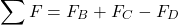# Axial Force – Calculation and Formula, Diagram, vs Other Forces

#### Engineering Most Popular

Axial force refers to a load whose line of action runs along the length of a structure or perpendicular to the structure’s cross-section. Moreover, the line of force goes through the center of gravity of the member’s cross-section. When this load tends to compress the member along its line of action, it is an axial compression load and carries a negative sign by convention. While if the load extends the member along its line of action, it is an axial tension load, carrying a positive sign.

In this article, you will understand the axial force calculation and formula, how to read its force diagram, and its behavior vs other types of forces.

### Calculation and Formula

When dealing with axial loading on a member, the three key parameters for carrying out calculations are the axial force, axial stress, and the cross-sectional area. So, for the area, what is of interest is the shape of the member perpendicular to the direction of the force. Generally, circular, or quadrilateral shapes are the most common load-carrying members. Although, triangular cross-sections and other shapes do exist for engineering structures. For the axial force, the directions of individual loads on the member sum together to find the resultant force magnitude and direction.

For example, the diagram above shows three axial forces acting on a cantilever beam at points B, C, and D. So, considering the impact of the forces on the cantilever, the force at D (FD) is a compressive force. While those at points B (FB) and C (FC) are tensile forces. Thus, the expression to obtain the resultant axial force on the beam (ƩF) is as follows:Hence, using the values from the diagram, the resultant force is 5 kN. As a result, it is a positive value, making it an axial tensile force. Therefore, for this structure to remain in equilibrium, the reaction at point ‘A’ should be equivalent to this resultant force. These two parameters – the resultant force (ƩF) and cross-sectional area (A) – can then be related to the stress (σ) on the cantilever as follows:## How to Read an Axial Force Diagram

An axial force diagram is a graphical representation of the axial force along the length of a member with an appropriate scale and sign convention. Generally, the x-axis represents the length of the member, while the y-axis quantifies the magnitude of force at each point along the structure. To understand how to read this diagram, it is important to know how to draw it, as the steps below highlight.

### Steps to Draw an Axial Force Diagram

• First, draw a free-body diagram of the structure.
• Then, to ensure uniformity between the free body diagram and the force diagram, place vertical lines at each point where there is a load change along the length of the member.
• After that, draw a horizontal axis representing the length of the member and the zero line of the force.
• Subsequently, select an appropriate scale for each axis and the sign convention for the y-axis. Generally, the positive y-axis values indicate tensile loads while the negative y-axis values indicate compressive loads.
• If a free end is present, then, start drawing the force diagram from it. Because this helps to evaluate the reaction values at any fixed end and can expose errors in any previous calculations.
• When drawing, a straight horizontal line represents the magnitude of the axial force along that section of the member. Then, at each loading point, which vertical lines indicate, move upwards for a tensile load, or downwards for compressive loads. If the load is a concentrated load, then the change is abrupt, with a vertical line indicating it. While for a distributed load, the change is gradual, with a diagonal line indicating it.
• Finally, as a check to confirm that the force diagram is correct, the force magnitude should always start from zero and end at zero.

The axial force diagram of the cantilever beam from the previous section serves as an example to better understand this concept.

### Cantilever Force Diagram Example

So, from the cantilever axial force diagram above, the magnitude of the force starts from 0 kN at the free end on the right side and gets to -7 kN. This indicates a compressive load at point ‘D’. Then, at point ‘C’, the tensile load of 4 kN causes an upward movement four places to -3 kN. Another tensile load of 8 kN at point ‘B’ takes the force value to 5 kN on the diagram. Finally, the compressive force of 5 kN from the reaction at the left of the cantilever takes the overall force value to 0 kN. Therefore, the diagram is accurate, and the member is in equilibrium.

## Behavior vs Other Types of Forces

Generally, it is common knowledge that the effects of axial forces on a structure are compression and tension. Moreover, there are other types of forces that provoke a variety of loading on a member. The following sections review some of these forces and compare them.

### Axial Force vs Normal Force

The normal force is the ground or surface reaction due to contact between two surfaces. Often, axial and normal forces are misjudged to be the same. However, there are a couple of differences between them.

### Axial Force vs Radial Force

It is possible to compare axial and radial forces if dealing with a cylindrical object. Or an object rotating about a fixed point, such as a shaft. Using this shaft example, the table below highlights some of the differences between the forces.